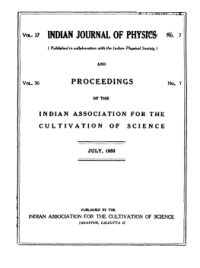About this item: 20.500.12592/b0pqj2

# Indian Journal of Physics and Proceedings of the Indian Association for the Cultivation of Science July 1953

1953

#### Summary

For g2=o.3 the numerical value of the cross section is of the order of m-22 cm2 and the total cross section increases as log EN Ep being the energy of the incident nucleon. [...] The third order matrix element H f i stands for H f J I (II ni2)(m2* 1 I i) „ (Ei — —E„ ) (2) Here the letters 1 In1 1112 and a denote the wave functions of the two nucleons in the final two successive intermediate and the initial states and Ei Em and stand for the total energies of the systems in the initial and the two successive intermediate states. [...] The coil in the combination was a 3o-henry choke and the capcitance of the condenser ranged from 0.0005 /AP to o.006 1LF so that the resonance frequency of the combination could be varied from 375 to 132o cycles/sec* and the equivalent impedance of the combination at its resonance frequency was very much higher than the input impedance of the amplifier The circuit diagram of the test circuit [...] INTRODUCTION The interpretation of the rotational structure of the bands of columbiutn oxide by the author led to the probability of assigning them to the electronic transition 4 As far as the author is aware there is no hand system till now investigated and ascribed to this transition. [...] These expressions enable the construction of the transition diagram for 4.1 ' shown in figure r. The other theoretical problem of the determination of the relative intensities of rotational lines in the expected branches that is the intensity factors has not been carried out so far.

Pages
48
SARF Document ID
sarf.120085